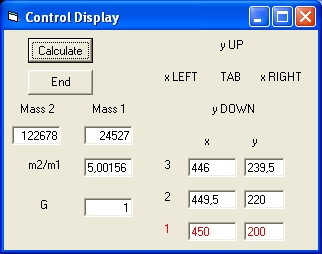## Visual Basic 5.0 Program: "VB Two Body"

The program VB Two_body uses the standard Newton's Law to calculate the masses of 2 objects based on observations written in Visual Basic 5.0.
Newton's Law assumes that the sum of all the forces of all objects is zero.
Newton's Law law describes that the force between two objects is "equal" to the mass m1 times the mass m2 of each object divided by the distance. Because this force acts twice but in opposite directions as such the sum is zero. Because this is true for all objects and all forces the sum of all forces is zero.

### VB Program: "VB Two_Body" Operation

The program uses two display or forms: Control Display, Simulation Display### Control Display

The Control Display contains 5 selection keys to modify the observed positions of object 1.
• The "TAB" key is used to select the observation you want to modify. The selected obsvervation has the colour RED.
• The "x RIGHT" key moves the point one unit to the right in the +X direction
• The "x LEFT" key moves the point one unit to the left in the -X direction
• The "y UP" key moves the point one unit in the up direction, +Y direction
• The "y DOWN " key moves the point one unit in the down, -Y direction
It is important to understand that X movement is independent that means when of one observation the X direction is changed the other observations are not changed.
For the Y movement this is different. See Operation Example For more details.
The Control Display contains the following parameters:
• G Constant of Gravity. This is the only parameter that can be changed. When you do that the calculated masses will change but this has no consequences for the simulation.
• Mass 1 This parameter contains the mass of object 1. Object 1 is initialy at the right. The value is calculated during the simulation. The selection keys modify the position of object 1.
• Mass 2 This parameter contains the mass of object 2. Object 2 is initialy at the left. The value is calculated during the simulation.
• m2/m1 This fraction is equal to the mass of object 2 divided by the mass of object 1.
• For each of the three observations and "x" value and an "y" value. The two values of selected observation is in red.
The VB program is controlled by two Commands:
• "Calculate" This Command is used to start the simulation.
• "End" This Command is used to End the execution of the Visual Basic program.

### Simulation Display

When you select the Calculate Command you will observe the Simulation Display. This display shows two circles.
• The outer circle is object 1. This is the smallest object. This is the observed object of which the positions are changed.
• The innner circle is object 2. This is the largest object. This is the object from which the observations are made.
The two green spots and the one red spot on the outer circle are the three observed positions.
When the TAB Key is selected you will observe that the position of the red spot changes.

The blue spot is the centre of gravity.

### Operation Example

• Select the Calculate pushbutton to start the simulation.
• With the "TAB" key select observation 3.
• With the "X left" key move the observation #3 to the left, to the value X = 444 and observe that the shape changes, that both masses change but ratio m2/m1 stays constant = 5,002
• With the "X right" key move the observation #3 to the right, to the value X = 446 and observe that only one of the "X" values changes. The "Y" values do not change.
• With the "Y up" key increase the "Y" value of observation #3 to Y = 243 and observe how the radius increases and that object 2 becomes more massif and the ratio increases to 5,876
• With the "Y down" key decrease the "Y" value of observation #3 to Y = 242 and observe that also the "Y" value of observation 1 decreases.
• With the "TAB" key select observation 1.
• With the "Y down" key decrease the "Y" value of observation #1 to Y = 189 and observe that also the "Y" value of observation 3 increases to 250,5. Ratio changes to 7.75
• With the "TAB" key select observation 3.
• With the "X left" key move the observation #3 to the left, to the value X = 436 and observe that the shape changes, the masses change but ratio m2/m1 stays constant = 7.75
• With the "TAB" key select observation 2.
• With the "Y down" key decrease the "Y" value of observation #2 to Y = 211 and observe that also the "Y" value of observation 1 decreases to 171 and the ration increases to 10.
• Select the End pushbutton to stop the simulation.

### VB Program: "VB Two_Body" source

In order to get the Visual Basic 5.0 source and the executable program use the following zip file:VB Two_Body.zip
The Zip files contains the following 6 files:
• (1) VB Newton C.vbp (2) VB Newton C.vbw (3) VB VB Newton C.frm (4) VB Newton C Display.frm (5) VB Newton C.bas and (6) VB Newton C.exe
The Visual Basic Executable program requires MSVDVM50.DLL: Msvdvm50.dll.zip

### Reflection

The most important message of the whole simulation is that the concept mass is of almost no physical importance to perform this simulation.
What is extremely important are the observations. Based on the observations the mass values are calculated using Newton's Law and the path of the two objects in the future can be calculated.